Related Articles

# Class 9 NCERT Solutions- Chapter 13 Surface Areas And Volumes – Exercise 13.6

• Difficulty Level : Medium
• Last Updated : 10 Mar, 2021

### Question 1. The circumference of the base of a cylindrical vessel is 132 cm and its height is 25 cm. How many liters of water can it hold? (1000 cm3 = 1l)

Solution: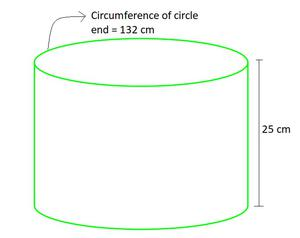Attention reader! All those who say programming isn't for kids, just haven't met the right mentors yet. Join the  Demo Class for First Step to Coding Coursespecifically designed for students of class 8 to 12.

The students will get to learn more about the world of programming in these free classes which will definitely help them in making a wise career choice in the future.

Given values,

Circumference of the base of a cylindrical = 132 cm

Height of cylinder (h)= 25 cm

Base of cylinder is of circle shape, having circumference = 2πr (r is radius)

Hence, 2πr = 132 cm

r =(taking π=)

r =r = 21 cm

So, volume of cylinder = πr2h

= 22/7 × 21 × 21 × 25                                 (taking π=)

= 34650 cm3

As, 1000 cm3 = 1 litre

34650 cm3× 34650

== 34.650 litres

### Question 2. The inner diameter of a cylindrical wooden pipe is 24 cm and its outer diameter is 28 cm. The length of the pipe is 35 cm. Find the mass of the pipe, if 1 cm3 of wood has a mass of 0.6 g.

Solution: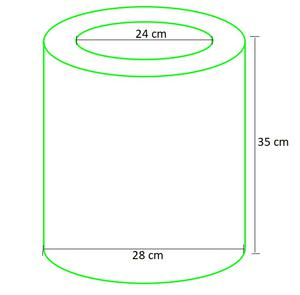Given values,

Inner radius of cylinder (r1)== 12 cm

Outer radius of cylinder (r2)== 14 cm

Height of cylinder (h)= 35 cm

So, volume used to make wood = volume of outer cylinder – volume of outer cylinder

= π(r22)h – π(r12)h

= π(r22 – r12)h× (142 – 122) × 35                     (taking π=)× (52) × 35

= 5720 cm3

As, 1 cm3 = 0.6 g

5720 cm3 = 0.6 × 5720 g

= 3432 grams

= 3.432 kg

### Which container has greater capacity and by how much?

Solution:

Let’s see each case,

(i) The shape of can is cuboid here, as having rectangular base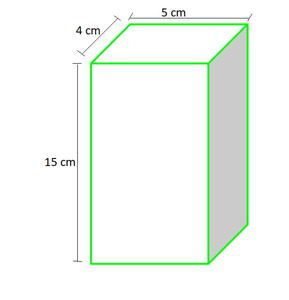Given values,

Length of can (l) = 5 cm

Width of can (b) = 4 cm

Height of can (h) = 15 cm

So, The amount of soft drink it can hold = volume of cuboid

= (l × b × h)

= 5 × 4 × 15 cm3

= 300 cm3

(ii)The shape of can is cylinder here, as having circular base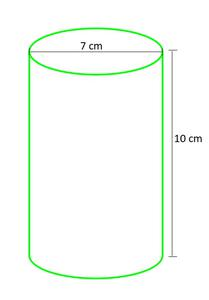Given values,

Radius of can (r) =cm

Height of can (h) = 10 cm

So, The amount of soft drink it can hold = volume of Cylinder

= (πr2h)× 10 cm3                                                  (taking π=)

= 385 cm3

Hence, we can see the can having circular base can contain (385 – 300 = 85 cm3) more amount of soft drinks than first can.

### Question 4. If the lateral surface of a cylinder is 94.2 cm2 and its height is 5 cm, then find

(ii) its volume. (Use π = 3.14)

Solution:

Given values,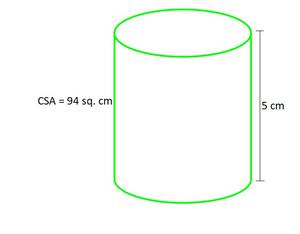Lateral surface of cylinder = 94.2 cm2

Height of cylinder (h) = 5 cm

Let’s see each case,

(i) So, the lateral surface is of rectangle shape whose

length = (circumference of base circle of cylinder) and width = height of cylinder

Let the base radius = r

Lateral surface = length × width

94.2 cm2 = (2πr) × h                         (circumference of circle = 2πr)

94.2 cm2 = (2 × 3.14 × r) × 5               (taking π = 3.14)

r =r = 3 cm

(ii) Given values,

Radius of cylinder (r)= 3 cm

So, the volume of cylinder = (πr2h)

= π × 3 × 3 × 5 cm3

= 3.14 × 3 × 3 × 5 cm3                    (taking π = 3.14)

= 141.3 cm3

### Question 5. It costs ₹2200 to paint the inner curved surface of a cylindrical vessel 10 m deep. If the cost of painting is at the rate of ₹20 per m2, find

(i) Inner curved surface area of the vessel,

(iii) Capacity of the vessel

Solution:

Given values,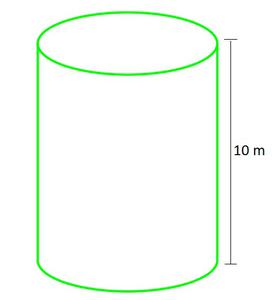Height of cylinder (h) = 10 m

Cost of painting rate = ₹20 per m2

Let’s see each case,

(i) For 1 m2 = ₹20

For lateral surface = ₹2200

So the lateral surface == 110 m2

(ii) Let the base radius = r

So as, Lateral surface = (circumference of base circle of cylinder) × height

110 m2= (2πr) × h

110 = (2 ×× r) × 10               (taking π=)

r =cm

r =cm

r = 1.75 cm

(iii) Volume of cylinder = (πr2h)× 10 cm3                  (taking π=)

= 96.25 cm3

### Question 6. The capacity of a closed cylindrical vessel of height 1 m is 15.4 liters. How many square meters of metal sheet would be needed to make it?

Solution:

Given values,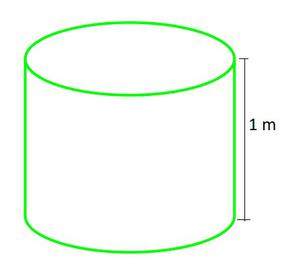Height of cylinder (h) = 1 m = 100 cm

Volume of cylinder (V) = 15.4 liters

As 1 liter = 1000 cm3

15.4 liters = 15.4 × 1000 cm3

V = 15,400 cm3

Volume of cylinder = (πr2h)

15,400 =× r2 × 100                                       (taking π=)

r2r2 = 49

r = √49

r = 7 cm

Surface area of a closed cylinder = (curve surface area + top and bottom circle) = 2πrh + (2 × πr2)

= 2πr (r+h)

= 2 ×× 7 × (7 + 100) cm2                                                   (taking π=)

= 2 × 22 × 107

= 4708 cm2

= 0.4708 m2

Hence, 0.4708 m2 of metal sheet would be needed to make it.

### Question 7. A lead pencil consists of a cylinder of wood with a solid cylinder of graphite filled in the interior. The diameter of the pencil is 7 mm and the diameter of the graphite is 1 mm. If the length of the pencil is 14 cm, find the volume of the wood and that of the graphite.

Solution:

So here pencil = (cylinder of wood + cylinder of graphite)

Given values,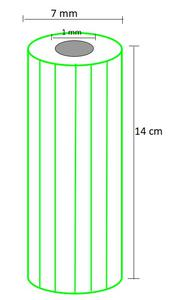Height of wood (and graphite) cylinder (h) = 14 cm = 140 mm

Radius of pencil (R)=mm

Radius of graphite (r)=mm

Volume of Graphite = (πr2h)× 140 mm3                                              (taking π=)

= 110 mm3

= 0.11 cm3

Volume of wood = Volume of pencil – Volume of graphite

= (πR2h) – (πr2h) = π(R2 – r2)h× (()2 – ()2) × 140 mm3                                      (taking π=)

= 22 × 20 × (–) mm3

= 22 × 20 × 12 mm3

= 5280 mm3

= 52.80cm3

### Question 8. A patient in a hospital is given soup daily in a cylindrical bowl of diameter 7 cm. If the bowl is filled with soup to a height of 4 cm, how much soup the hospital has to prepare daily to serve 250 patients?

Solution:

So here Volume of soup for each patient = Volume of cylinder.

Given values,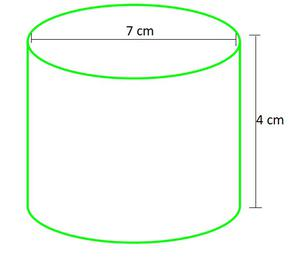Height of cylinder (h) = 4 cm

Radius of cylinder (r)=cm

Volume of Cylinder = (πr2h)×× 4 cm3                                             (taking π=)

= 154 cm

Volume of soup for 250 patient = 250 × Volume of cylinder.

= 250 × 154

= 38,500 cm3

Hence, 38,500cm3 soup is needed daily to serve 250 patients.

My Personal Notes arrow_drop_up